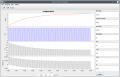# Buck converter modelling simulation - differential equations

#### ag-123

Joined Apr 28, 2017
276
I'm trying to model the buck converter. So here is a model schematic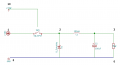to simplify the model, i divided it into 'on' phase and 'off' phase as follows:
on phase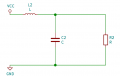derived equation: Vin here is the VCC symbol, to denote the driving input. Vo the output voltage is the voltage across C and R (load)
$V_{in} = L C \frac{d^2 V_o}{dt^2} + \frac{L}{R} \frac{d V_o}{dt} + V_o \\ where V_o = V_r = V_c$

"off" phase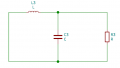$L C \frac{d^2 V_o}{dt^2} + \frac{L}{R} \frac{d V_o}{dt} - V_o = 0$

so I took the equations, write a little program and simulated it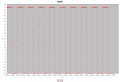erm ok, it doesn't seemed to be a buck converter at all. The expected output is a DC output across R.
How the simulation is done is by using numerical integration Runge–Kutta methods. Those equations above are 2nd order ODE, the 'tools' used are those of apache commons math

I've attached the derivations of the ODE in the post attachments here
The problem is, are the derivations or equations incorrect?

#### Attachments

• 81.2 KB Views: 4
Last edited:

#### ag-123

Joined Apr 28, 2017
276
it seemed possible that there could be numerical problems as well.
when I run the simulations at 1 khz 50% duty cycle, integration time steps at 10 k samples per sec
it gives 4.6v as peak output voltages, they are literally 'square' waves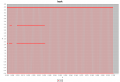But if I run the same simulation 1khz 50% duty cycle at integration time steps 100 k samples per sec the output voltages drops to 0.05v !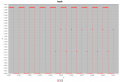now the 'square' waves are more apparent as the integration time steps is 100 times smaller, prior it is 10 times smaller vs the PWM frequencies. this one gives 0.05v, which the coarser integration time gives 4.6v. Vin is 10v.
But there could be other issues as well, e.g. the capacitor don't seem to have an effect to keep voltages higher. ODE is incorrect?
tried various frequencies 1k, 10k and even 100k, numerical problem persists. But then it is still 'square' waves rather than DC output.

Last edited:

#### Papabravo

Joined Feb 24, 2006
20,603
I think you are missing a couple of things here:
1. A typical switching frequency for a buck converter would be in the range of 50-500 kHz. There are some designs that go higher, but very few that go lower.
2. An unexpected decrease in the output voltage is a result of operating in DCM (Discontinuous Conduction Mode). This is where the current in the inductor drops to zero and the capacitor tries to maintain the output voltage until the switch is turned back on. The opposite of DCM is CCM (Continuous Conduction Mode) where you do not allow the inductor current to drop to zero.
3. Both the inductor and the capacitor have to be properly sized to guarantee CCM operation.
Here is an example of an open loop buck converter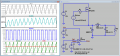The operating frequency is 200 kHz (5usec period). The reference voltage is set a bit higher than the desired 5V output to account for losses in the MOSFET and the Schottky diode. You can see the current ripple of about 240 mA around the average value of 5 amperes. You can also see the voltage ripple of about 75 mV P-P which is about 1.5%. Notice also the parasitic components, the DCR for the inductor and the ESR for the capacitor.

Try using my component values and switching frequency with your model and see if the results are consistent. My duty cycle is 5.4V/10V = 54%. V(pwm) = 15V, and the switch M1 will be ON, whenever V(Ramp) < V(Ref)

Last edited:

#### ag-123

Joined Apr 28, 2017
276
Thanks Papabravo !

fixed a bug in the numerical integration step
1 khz 50% duty cycle 10k samples per sec integration steps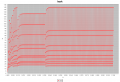now it looked like a boost converter :/
There are apparently other bugs for all that spiky voltages. I'd need to debug it along.
for a start, that exponentially rising voltages seemed to look more like a buck (or more like boost) converter.
But the extreme spikes is unlikely to be seen in a real buck converter.

Last edited:

#### Papabravo

Joined Feb 24, 2006
20,603
In my post, I showed the waveforms after the startup transients had subsided. Normally we get the steady state working first, then tackle the transient behavior at startup and during a load transients after we have closed and compensated the loop.

#### ag-123

Joined Apr 28, 2017
276
Last edited:

#### ag-123

Joined Apr 28, 2017
276
now this looks like a buck converter, this again is done with only the "on phase" ODE
1 khz 50% duty cycle 100k samples per sec integration steps
C = 100 uF, L = 100 uH, R = 1k, Vin = 10 v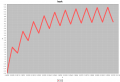it turns out for Runge–Kutta methods timesteps matter, if it isn't sufficiently fine, the computations results in spiky oscillations, as seen earlier. the sample frequency 100 k sps is 100 times of the PWM frequency 1 khz.
This "sawtooth" is 'expected' as my PWM frequencies is low for the L and C values provided.
the 2nd ODE seemed redundant, and I probably used the incorrect initial values that result in that 'boost' calcs.

Last edited:

#### Papabravo

Joined Feb 24, 2006
20,603
ODE solvers apparently are not comfortable with switching systems where the underlying circuitry changes but remains continuous and piecewise linear. At the "corners", derivatives become indeterminate. It is almost like dealing with stochastic functions that are everywhere continuous, but nowhere differentiable. That they are made up exclusively of "corners". I'm not sure if any standard ODE solver is a useful approach to modeling or understanding DC-DC converters. I know the LTspice engine will adjust the timestep based on the local derivative at the point of solution. On very fast edges it can throw a "timestep too small" error. For example, an actual step function with a slope (derivative) in excess of 1E308 would be problematical. I typically use 10nsec for rise and fall times in my simulations without many difficulties.

One more thing: your "OFF" circuit does not include the voltage drop across the diode. While this number is usually small with respect to the output voltage, it is a function of the current circulating during the OFF state.

Last edited:

#### ag-123

Joined Apr 28, 2017
276
thanks Papabravo, at the moment, this rather simple model is adequate to 'get started' doing some 'sensitivity' studies with the various L, C and R values.
I'd agree about the 'missing' diode, in the off-state equation.
For now, it seemed the "on state" equation is correct. I'd guess the "off state" would still be necessary to model the interaction with the diode. Or i'd perhaps work that into the "on state" model. This is deliberately simplified as when more components are added, the ODE becomes pretty much more complicated and harder to tell if it is after all correct.
it seemed useful to aim at the LC resonant frequencies for the PWM frequencies, while i've not really worked that out, it seem to be around the 10s of khz for the 100 uH 100 uF combinations. Real ones would have resistances and there is in addition a diode which is not accounted.
Too low a frequency results in the "sawtooth" voltages.

#### Papabravo

Joined Feb 24, 2006
20,603
thanks Papabravo, at the moment, this rather simple model is adequate to 'get started' doing some 'sensitivity' studies with the various L, C and R values.
I'd agree about the 'missing' diode, in the off-state equation.
For now, it seemed the "on state" equation is correct. I'd guess the "off state" would still be necessary to model the interaction with the diode. Or i'd perhaps work that into the "on state" model. This is deliberately simplified as when more components are added, the ODE becomes pretty much more complicated and harder to tell if it is after all correct.
it seemed useful to aim at the LC resonant frequencies for the PWM frequencies, while i've not really worked that out, it seem to be around the 10s of khz for the 100 uH 100 uF combinations. Real ones would have resistances and there is in addition a diode which is not accounted.
Too low a frequency results in the "sawtooth" voltages.
I think that you will find a SPICE simulator more suited to your purpose, and as a bonus you'll be able to focus on your task without worrying about the underlying mathematics. Your results will be based on actual component models with parasitics.

#### ag-123

Joined Apr 28, 2017
276
thanks, Papabravo SPICE works well. However, in context, i'm intending to build one out of an MCU.
As the PWM frequencies and duty cycles are then freely selectable, I'm simulating this from a control perspective.
It appears from experiments (i.e. this simulation) that for buck converters very little 'control' is needed.
all it takes is fixed frequencies, and duty cycle determines the voltages.

I'm able to simulate some kind of "discontinuous conduction mode"
10 khz 1% duty cycle 100k samples per sec integration steps
L 100 uH C 100 uF R 1k Vin 10v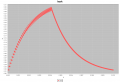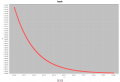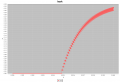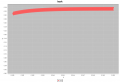I'm not too sure if such artefacts will occur in real circumstances.

#### ag-123

Joined Apr 28, 2017
276
Last edited:

#### ag-123

Joined Apr 28, 2017
276
In any case here is it:
https://github.com/ag88/jbuckconv
requires Java 11 to run it.
you can use the release
https://github.com/ag88/jbuckconv/releases/tag/v0.1.0
saves the step of building it

practically, if anyone is willing to derive those ODE and if the Runge–Kutta integrator from Apache Commons Math can handle it, you could simulate more elaborate circuits. This would require editing the codes to insert the ODE.
The other benefit is you can use any sort of driving functions you prefer since this is just an ODE.
In this instance, the square waves is provided by the Vin() method in BuckODE.java, you could code it in other ways to make driving functions you prefer, PID etc.

#### ag-123

Joined Apr 28, 2017
276
I'm now experimenting with the 'missing' diodeSo the "off state" circuit looks like this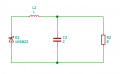It turns out there is something we'd typically "ignore"
Code:
DEBUG BuckODE compute 2nd deriv - t : 5.0E-5, y: 0.24730010141055617, yDot: 0.0
DEBUG BuckODE vin - Vin: 10.0, t: 0.00005, cycle: 0.5, fcycle: 0.5 <- it is still "on state here"
DEBUG BuckODE compute 2nd deriv - yDDot: 9.752699898589444E8
switches to off state at this moment

Code:
DEBUG BuckODE compute 2nd deriv - t : 5.5E-5, y: 0.24730010141055617, yDot: 4876.349949294721
DEBUG BuckODE vin - Vin: 0.0, t: 0.00006, cycle: 0.55, fcycle: 0.55
^ over here the Vout - is 0.2473 volts (denoted by y)
but the $$\frac{d V_{out}}{dt}$$ - yDot is a pretty high value (the 'velocity' of the change in voltages)

During that mosfet (or transistor) switching transient, from high to low. This is abruptly changed. In effect, the rising capacitor voltages is blocked, and literally, the pretty high 'accelerations' in voltages
$$\frac{d^2 V_{out}}{dt^2}$$, which reads $$yDDot: 9.752699898589444E8$$ just before the switching transient is also abruptly halted. The initial conditions at this point completely changed.

Code:
DEBUG BuckODE Vd - Vd : 0.1223044622115175
DEBUG BuckODE compute 2nd deriv - yDDot: -3.696045636220737E7
DEBUG BuckODE compute 2nd deriv - t : 5.5E-5, y: 0.24730010141055617, yDot: -184.80228181103683
DEBUG BuckODE vin - Vin: 0.0, t: 0.00006, cycle: 0.55, fcycle: 0.55
DEBUG BuckODE Vd - Vd : 0.0
DEBUG BuckODE compute 2nd deriv - yDDot: -2.4728162118237507E7
On a side note, the ODE now looks more complicated
$\begin{split} L\frac{d}{dt} ( C \frac{dV_o}{dt} + \frac{V_o}{R} ) + V_o + V_D & = 0 \\ L C \frac{d^2 V_o}{dt^2} + \frac{L}{R} \frac{d V_o}{dt} + V_o + V_D = 0 \\ L C \frac{d^2 V_o}{dt^2} + \frac{L}{R} \frac{d V_o}{dt} + V_o + n V_T \ln{(\frac{I_D}{I_s} + 1)} = 0 \\ L C \frac{d^2 V_o}{dt^2} + \frac{L}{R} \frac{d V_o}{dt} + V_o + n V_T \ln{(\frac{C \frac{dV_o}{dt} + \frac{V_o}{R}}{I_s} + 1)} = 0 \\ \end{split}$
This is a complicated way of saying voltage over inductor + voltage over load and capacitor + voltage over diode = 0. :/
The last term being that of the diode. It is actually the Shockley diode equation, the parameters are borrowed from SPICE models.

There are apparently limitations with all that integraion, Vd - diode voltage isn't likely to be zero. As $$\frac{d V_{out}}{dt}$$ is negative, this actually produce an error, negative logarithms is undefined. So I initialised diode voltage to zero to work around the problems. I'm thinking, I'd set this "velocity" as zero, as the capacitor just about starts to discharge.

That probably explains the incorrect earlier "boost" results. I'm still trying to figure out what might be appropriate for the initial conditions during switching.

Last edited:

#### ag-123

Joined Apr 28, 2017
276
Last edited: